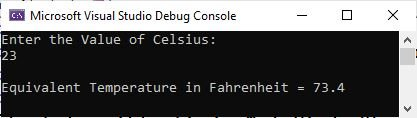# C# program to convert celsius to fahrenheit

This post is published to provide the tutorial that shows how we can use a C# program to convert temperature from celsius to fahrenheit.

## Simple C# code to convert celsius to fahrenheit

Let me create a simple C# program that converts Celsius to Fahrenheit. But before writing the code, let me tell you the formula that is used to convert celsius to fahrenheit.

`F = (C * 1.8) + 32`

In this formula, F indicates Fahrenheit, whereas C indicates Celsius. Now let's create the program to implement this formula.

```float c = 37;
float f = (float) (c * 1.8) + 32;

Console.WriteLine(f);```

The output should exactly be:

`98.6`

In the above example, the (float) before (c * 1.8) is used to do typecasting. I have already discussed this topic. You can also use the double type, which I am going to use in an upcoming example.

## C# code to convert celsius entered by the user to fahrenheit

Now let me create another example that does the same job as the previous example. The only difference is that this program allows the user to enter the value of Celsius at run-time of the program.

```double celsius, fahrenheit;

Console.WriteLine("Enter the Value of Celsius: ");

fahrenheit = (celsius * 1.8) + 32;

Console.WriteLine("\nEquivalent Temperature in Fahrenheit = " + fahrenheit);```

Now the sample run with user input of 23 as temperature in celsius is shown in the snapshot given below.C# Online Test

« Previous Program CodesCracker.com »